# BCA Assay Trial 2

## If at first you don’t succeed, trial trial again.

Happy #MicroplateMonday! With a working microplate reader available to us, we proceeded (again) with the BCA Assay. We followed the protocol generated during our last attempt. The only difference is that I broke the bottom of microplate well E9. Therefore, sample O07 only had two replicates (E7 and E8).

#### Some photos from our lab work: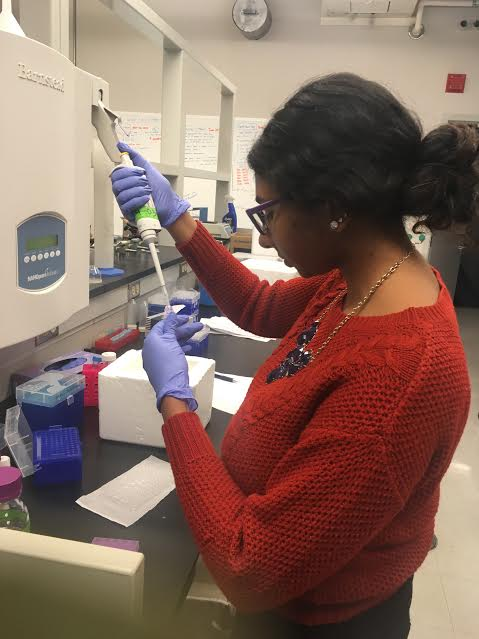Figure 1. Here I am pipetting 10 µL of my sample for the microplate.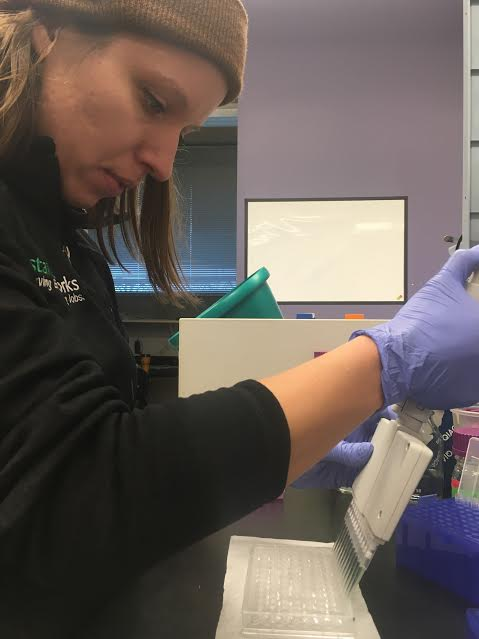Figure 2. Laura using a multipipetter to add 200 µL of our BCA working reagent to each well.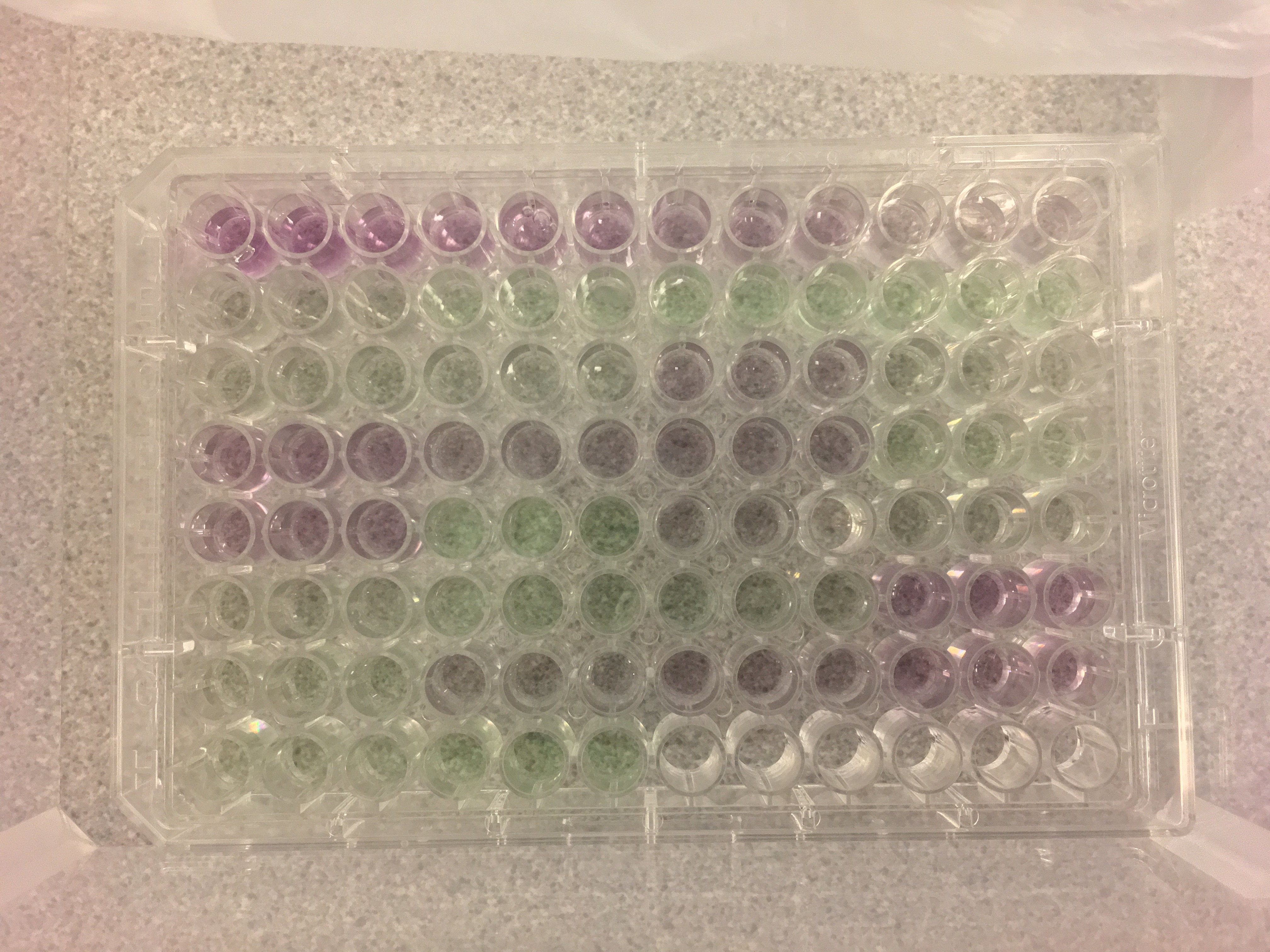Figure 3. Our completed microplate! Well A1 is in the top left corner. Microplate contents specified here.

#### Once in the Genome Sciences Building, we did the following:

• Covered plate with film to prevent solutions from evaloprating
• Using an Eppendorf ThermoMixer C, we incubated our plate for 30 minutes at 37ºC
• Vortexed plate to mix solutions thoroughly
• Centrifuged plate for approximately one minute at 1500 rpm using Beckman-Couter centrifuge
• Used Labsystems Multiskan MCC/340 to read plate at 540 nm
• Opened Ascent Software Version 2.6 on accompanying computer

#### Calculations

In order to start the Mini-Trypsin digestion, we need to know the volume of our sample that contains 30 µg of protein. To calculate this volume, we used the following steps.

• Calculate average wavelength for each standard and unknown samples using wavelength for each replicate
• Substract average absorbance for the blank standards from the average absorbance of the remaining standards and unknown samles
• This is the blank-corrected absorbance
• Create a scatterplot for the standards
• y-axis: BSA concentration (µg/µL)
• x-axis: blank-corrected absorbances (nm)
• Display equation and R-squared value
• Using the trendline equation in the scatterplot, calculate protein concentration from absorbance
• Multiply each concentration by three
• We do this because we diluted our sample 1:2 with 50 mM NH4HCO3 in 6M Urea
• For each sample, calculate volume (µL) needed for 30 µg protein
• Need 100 µL to start Mini-Trypsin digestion
• Calculate how much 50 mM NH4HCO3 in 6M Urea needed for each sample to reach 100 µL volume
• 100 µL total volume - volume for 30 µg protein

The scatterplot I generated and a table of calculations are below: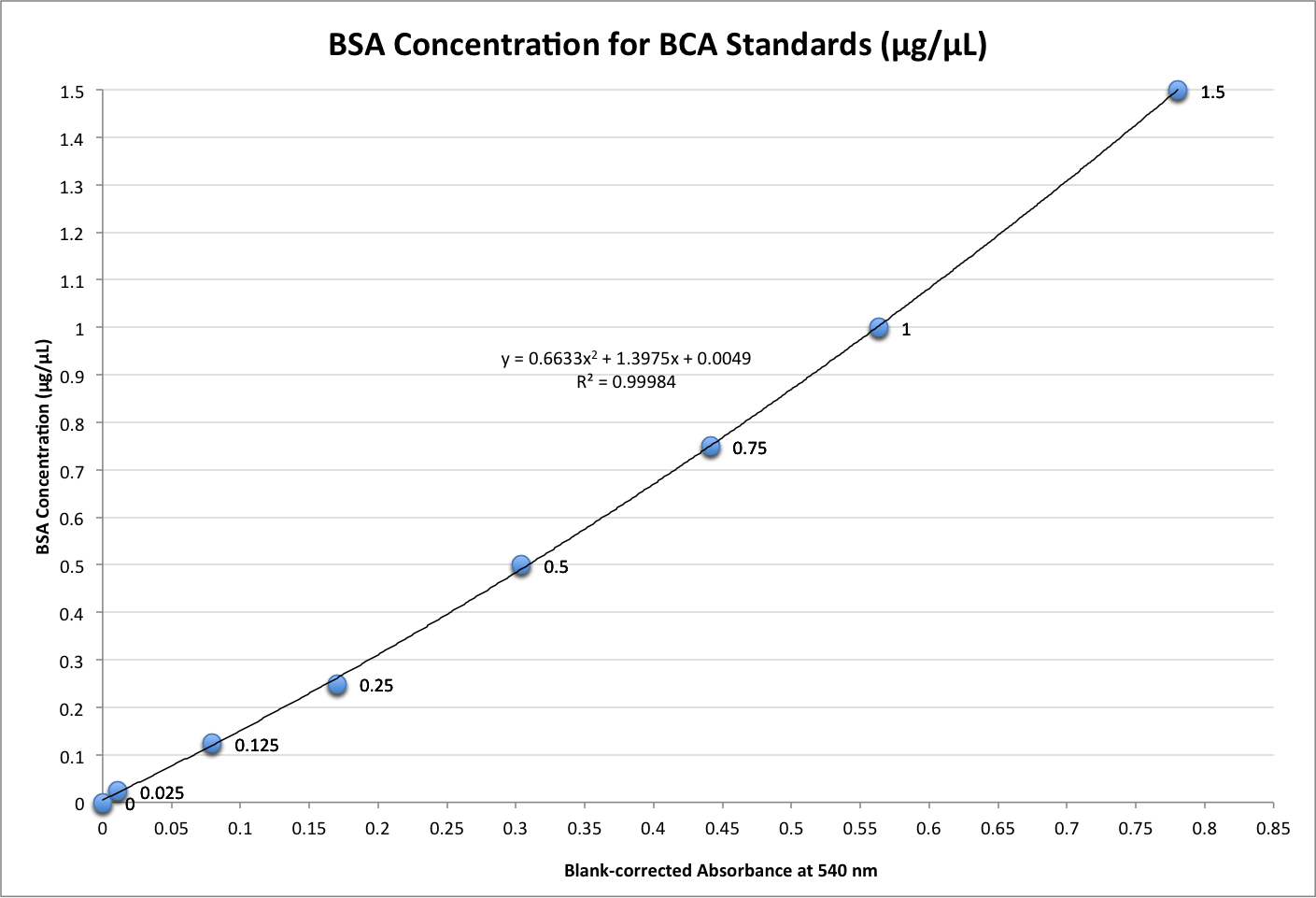Figure 4. Scatterplot of BSA concentration (µg/µL) versus blank corrected absorbances (nm). The trendline equation, y = 0.6633x2 + 1.3975x + 0.0049, was used to calculate protein concentration from absorbances.

Table 1. Calculations for each sample. Volume of samples and 50 mM NH4HCO3 in 6M Urea needed for 100 µL total volume based on having 30 µg of protein. Note: “O124” should read “0127” under the column “Sample”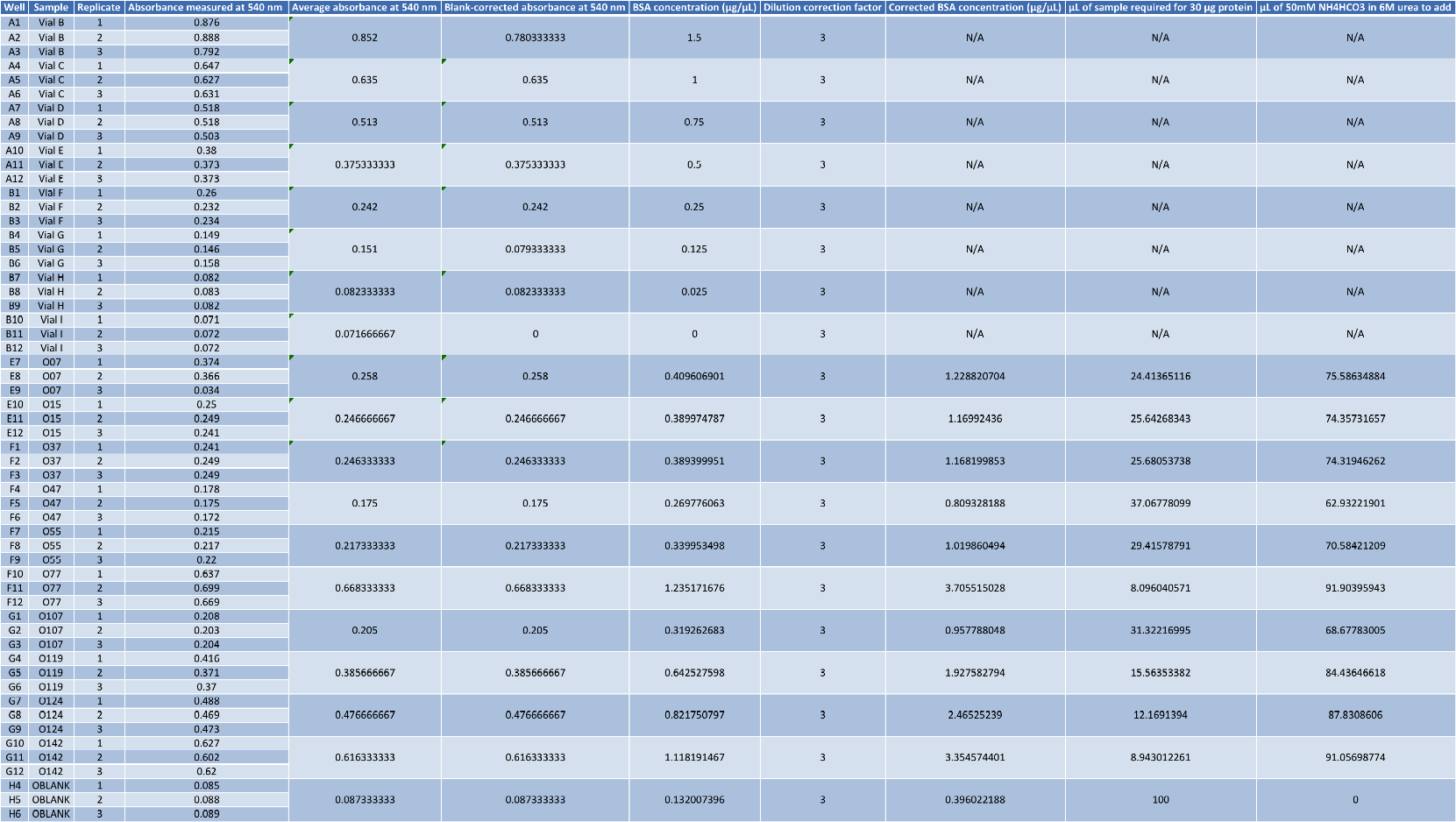Check back Tuesday for our Mini-Trypsin digestion! #TrypsinTuesday #MakeEverythingAHashtag

Written on December 12, 2016